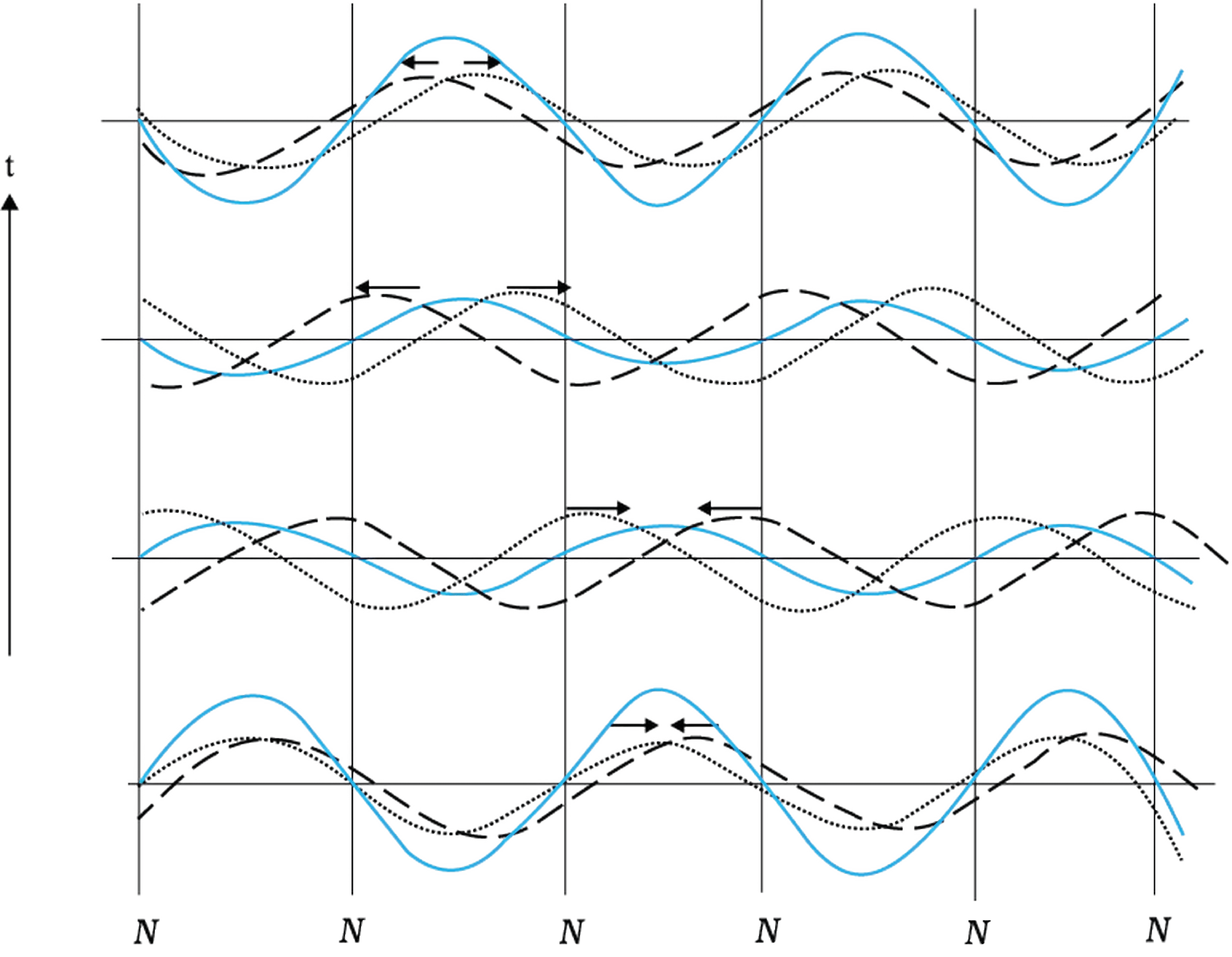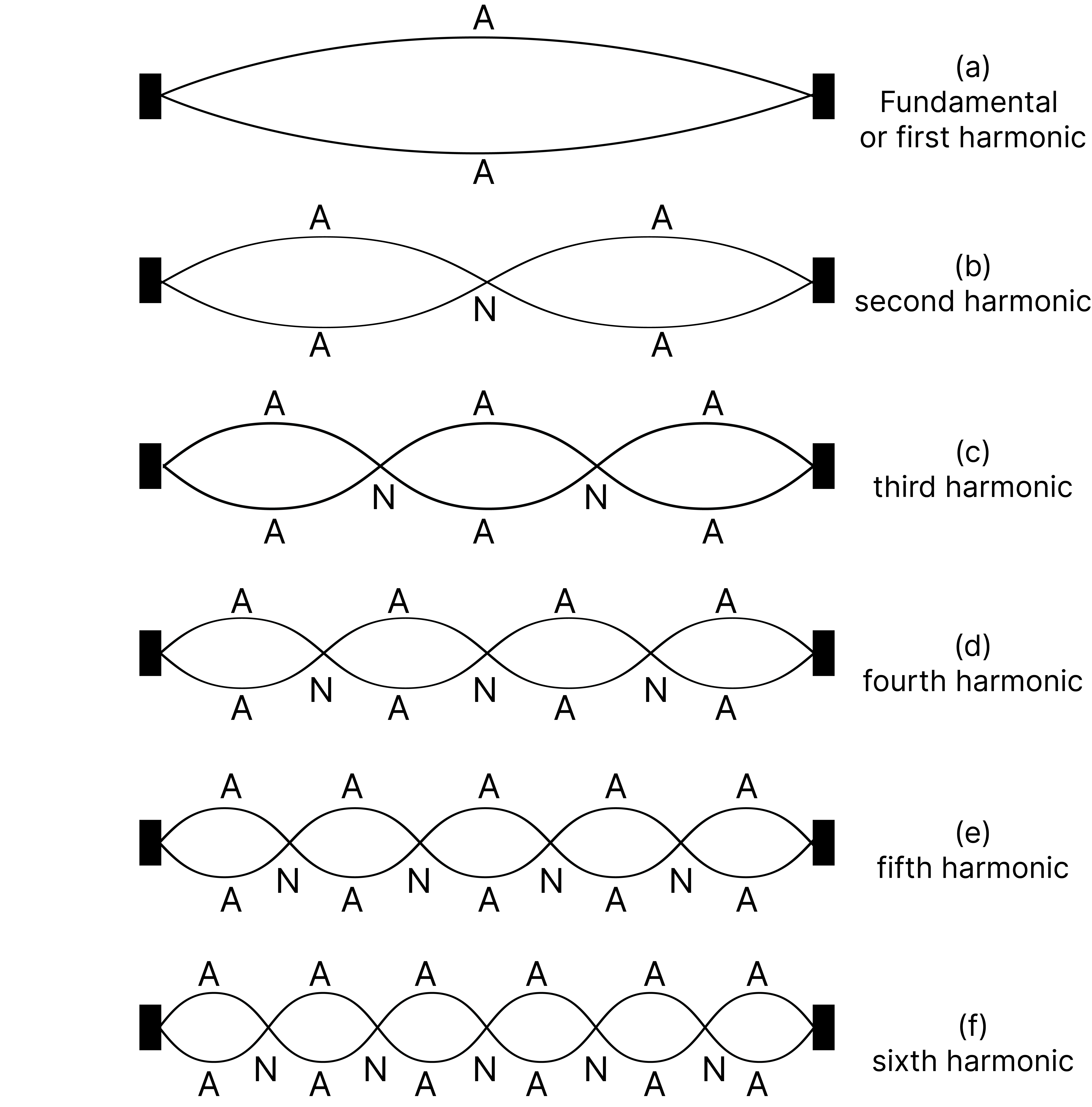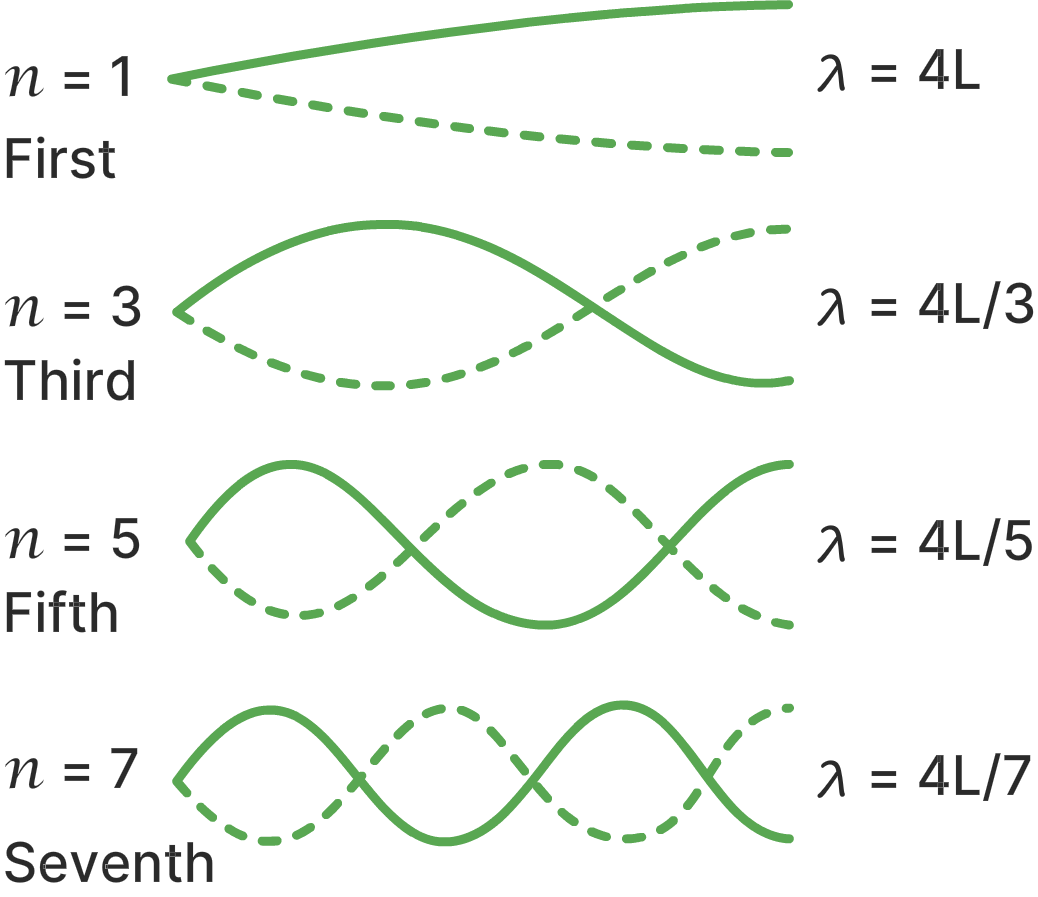Demonstration of Standing Waves using a Spring

# Objective

To demonstrate standing waves with a spring.

# Theory

## Stationary waves

Consider a string tied to a hook. A pulse or wave starts from the free end. When a wave meets a rigid boundary, it gets reflected with a phase change of Pi. The superposition of the incident wave and reflected wave results in a steady wave pattern on the string. These wave patterns are called stationary waves or standing waves.Figure 1: Stationary waves arising from superposition of two harmonic waves travelling in opposite direction. Nodes and antinodes remain fixed at all times

## Definition of standing waves

When two sinusoidal waves of the same amplitude and wavelength travel in opposite directions along a stretched string, their interference with each other produces a standing wave or stationary wave.

Consider an incident wave is travelling in the positive direction x-axis.

𝑦₁(𝑥,𝑡) = 𝑎 𝑠𝑖𝑛(𝑘𝑥 - ω𝑡)

The reflected wave is travelling in the negative direction of the x-axis.

𝑦₂(𝑥,𝑡) = 𝑎 𝑠𝑖𝑛(𝑘𝑥 + ω𝑡)

The resultant wave by the superposition principle is

𝑦(𝑥,𝑡) = 2𝑎 𝑠𝑖𝑛 𝑘𝑥 𝑐𝑜𝑠 ω𝑡

The amplitude of the resultant wave changes from one point to another point. But all parts of the string oscillate with the same time period.

Nodes- These are points in the wave where the amplitude in the wave is minimum.

Antinodes –These are points in the wave where the amplitude in the wave is maximum.

The wave pattern is stationary. The locations of nodes and antinodes do not change in resultant waves. For this reason, the resultant wave is referred to as stationary.

Normal modes- The system vibrates by a set of natural frequencies. These are normal modes. Boundary conditions constrain possible frequencies of vibrations of system.

• Consider a string with both fixed ends. Both ends of the strings are nodes.

The possible wavelength of stationary waves

λ = 2𝐿/𝑛 ; 𝑛 = 1, 2, 3,......

with corresponding frequencies

𝛎 = nv/2L; 𝑛 = 1, 2, 3,......

L - length of string

n - order of harmonic

v - speed of wave

Lowest natural frequency (n=1) is called fundamental frequency.

n = 2 - Second harmonic

n = 3 - Third harmonic.Figure 2: The first six harmonics of vibration of a stretched string fixed at both ends

• Consider a string with one fixed end and one free end. Here, the free end of the string is antinode. The fixed end of the string is the node.

The possible wavelength of stationary waves

λ = 4𝐿/𝑛 ; 𝑛 = 1, 3, 5,......

with corresponding frequencies

𝛎=v/λ= nv/4LFigure 3: Harmonics of vibrations of a stretched string with one free end and one fixed end

# Learning Outcomes

• Students understand how standing waveforms on the spring.
• Students understand how standing waves change when boundary conditions are changed.
• Students understand how time periods of different harmonics are related.